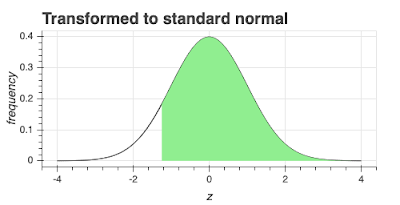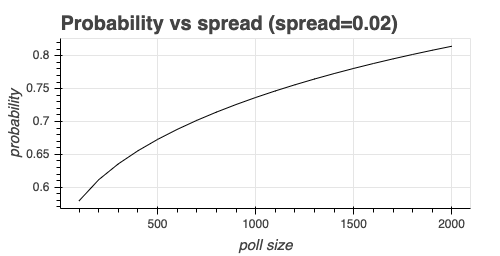# Polls to probabilities

How likely is it that your favorite candidate will win the election? If your candidate is ahead of their opponent by 5%, are they certain to win? What about 10%? Or if they're down by 2%, are they out of the race? Victory probabilities are related to how far ahead or behind a candidate is in the polls, but the relationship isn't a simple one and has some surprising consequences as we'll see.

# Opinion poll example

Let's imagine there's a hard-fought election between candidates A and B. A newspaper publishes an opinion poll a few days before the election:

• Candidate A: 52%
• Candidate B: 48%
• Sample size: 1,000

Should candidate A's supporters pop the champagne and candidate B's supporters start crying?

# The spread and standard error

Let's use some standard notation. From the theory of proportions, the mean and standard error for the proportion of respondents who chose A is:

$p_a = {n_a \over n}$ $\sigma_a = { \sqrt {{p_a(1-p_a)} \over n}}$

where $$n_a$$ is the number of respondents who chose A and $$n$$ is the total number of respondents. If the proportion of people who answered candidate B is $$p_b$$, then obviously, $$p_a + p_b = 1$$.

Election probability theory usually uses the spread, $$d$$, which is the difference between the candidates: $d = p_a - p_b = 2p_a - 1$ From statistics theory, the standard error of $$d$$  is: $\sigma_d = 2\sigma_a$ (these relationships are easy to prove, but a bit tedious, if anyone asks, I'll show the proof.)

Obviously, for a candidate to win, their spread, $$d$$, must be > 0.

# Everything is normal

From the central limit theorem (CLT), we know $$p_a$$ and $$p_b$$ are normally distributed, and also from the CLT, we know $$d$$ is normally distributed. The next step to probability is viewing the normal distribution for candidate A's spread. The chart below shows the normal distribution with mean $$d$$ and standard error $$\sigma_d$$.As with most things with the normal distribution, it's easier if we transform everything to the standard normal using the transformation: $z = {(x - d) \over \sigma_d}$ The chart below is the standard normal representation of the same data.The standard normal form of this distribution is a probability density function. We want the probability that $$d>0$$ which is the light green shaded area, so it's time to turn to the cumulative distribution function (CDF), and its complement, the complementary cumulative distribution function (CCDF).

# CDF and CCDF

The CDF gives us the probability that we will get a result less than or equal to some value I'll label $$z_c$$. We can write this as: $P(z \leq z_c) = CDF(z_c) = \phi(z_c)$ The CCDF is defined so that: $1 = P(z \leq z_c) + P(z > z_c)= CDF(z_c) + CCDF(z_c) = \phi(z_c) + \phi_c(z_c)$ Which is a long-winded way of saying the CCDF is defined as:  $CCDF(z_c) = P(z_c \gt 0) = \phi_c(z_c)$

The CDF is the integral of the PDF, and from standard textbooks: $\phi(z_c) = {1 \over 2} \left( 1 + erf\left( {z_c \over \sqrt2} \right) \right)$ We want the CCDF,  $$P(z > z_c)$$, which is simply 1 - CDF.

Our critical value occurs when the spread is zero. The transformation to the standard normal in this case is: $z_c = {(x - d) \over \sigma_d} = {-d \over \sigma_d}$ We can write the CCDF as: $\phi_c(z_c) = 1 - \phi(z_c) = 1- {1 \over 2} \left( 1 + erf\left( {z_c \over \sqrt2} \right) \right)\$ $= 1 - {1 \over 2} \left( 1 + erf\left( {-d \over {\sigma_d\sqrt2}} \right) \right)$ We can easily show that: $erf(x) = -erf(-x)$ Using this relationship, we can rewrite the above equation as: $P(d > 0) = {1 \over 2} \left( 1 + erf\left( {d \over {\sigma_d\sqrt2}} \right) \right)$

What we have is an equation that takes data we've derived from an opinion poll and gives us a probability of a candidate winning.

# Probabilities for our example

For candidate A:

• $$n=1000$$
• $$p_a = {520 \over 1000} = 0.52$$
• $$\alpha_a = 0.016$$
• $$d = {{520 - 480} \over 1000} = 0.04$$
• $$\alpha_d = 0.032$$
• $$P(d > 0) = 90\%$$

For candidate B:

• $$n=1000$$
• $$p_b = {480 \over 1000} = 0.48$$
• $$\alpha_b = 0.016$$
• $$d = {{480 - 520} \over 1000} = -0.04$$
• $$\alpha_d = 0.032$$
• $$P(d > 0) = 10\%$$

Obviously, the two probabilities add up to 1. But note the probability for candidate A. Did you expect a number like this? A 4% point lead in the polls giving a 90% chance of victory?

# Some consequences

Because the probability is based on $$erf$$, you can quite quickly get to highly probable events as I'm going to show in an example. I've plotted the probability for candidate A for various leads (spreads) in the polls. Most polls nowadays tend to have about 800 or so respondents (some are more and some are a lot less), so I've taken 800 as my poll size. Obviously, if the spread is zero, the election is 50%:50%. Note how quickly the probability of victory increases as the spread increases.What about the size of the poll, how does that change things? Let's fix the spread to 2% and vary the size of the poll from 200 to 2,000 (the usual upper and lower bounds on poll sizes). Here's how the probability varies with poll size for a spread of 2%.Now imagine you're a cynical and seasoned poll analyst working on candidate A's campaign. The young and excitable intern comes rushing in, shouting to everyone that A is ahead in the polls! You ask the intern two questions, and then, like the Oracle at Delphi, you predict happiness or not. What two questions do you ask?

• What's the size of the poll?

# What's missing

There are two elephants in the room, and I've been avoiding talking about them. Can you guess what they are?

All of this analysis assumes the only source of error is random noise. In other words, there's no systemic bias. In the real world, that's not true. Polls aren't wholly based on random sampling, and the sampling method can introduce bias. I haven't modeled it at all in this analysis. There are at least two systemic biases:

• Pollster house effects arising from house sampling methods
• Election effects arising from different population groups voting in different ways compared to previous elections.

Understanding and allowing for bias is key to making a successful election forecast. This is an advanced topic for another blog post.

The other missing item is more subtle. It's undecided voters. Imagine there are two elections and two opinion polls. Both polls have 1,000 respondents.

Election 1:

• Candidate A chosen by 20%
• Candidate B chosen by 10%
• Undecided voters are 70%
Election 2:

• Candidate A chosen by 55%
• Candidate B chosen by 45%
• Undecided voters are 0%